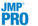Publication date: 07/30/2020

##Random Coefficients

For each random effect, the Mixed Models personality provides a report showing estimated coefficients and a report showing the matrix of covariance estimates. Each row of the coefficients report corresponds to one level of the random effect. The row shows all coefficient estimates associated with that level of the random effect. The random coefficient estimates are used in conjunction with fixed effect estimates to create predictions for any specific level of the random effect.

##Random Effects Predictions

For each random effect in the model, this report gives an estimate known as the best linear unbiased predictor (BLUP), its standard error, and a Wald-based confidence interval.

Estimation of the standard errors requires calculation of the BLUP covariance matrix, which can be time-intensive. If the calculation time is noticeable, a progress bar appears.